﻿ A Preprocessing Method for Improved Compression of Digital ImagesPublications are Open
Access in this journal
Article Versions
Export Article
• Normal Style
• MLA Style
• APA Style
• Chicago Style
Research Article
Open Access Peer-reviewed

### A Preprocessing Method for Improved Compression of Digital Images

Biju Bajracharya , David Hua
Journal of Computer Sciences and Applications. 2018, 6(1), 32-37. DOI: 10.12691/jcsa-6-1-4
Published online: June 04, 2018

### Abstract

Image compression methods are used to efficiently reduce the volume of image transmission and storage. Pre-processing of images are done to remove spurious noise or unwanted detail from an image to improve the compression performance. This paper proposes a preprocessing method for image compression based on ±K adjustment to a pixel value that enables high compression ratio without losing visual quality. Visual quality of an image was measured using peak signal to noise ratio (PSNR) as a metric. This method was designed based on mapping table constructed from histogram to identify pixels that hinder high compression ratios. These identified pixels were adjusted by ±k values which yielded higher compression ratios. The designed method had six levels of operations. Higher levels retained most of their original pixel values, thus maintaining higher PSNR values at lower compression ratios. Lower levels achieved higher compression ratios by adjusting more pixels (lower PSNR values). A value of ±1 was used for retaining better original information, while ±2, ±3 and higher were used for higher compression ratios. Preprocessed and non-preprocessed grey scale images were compressed using popular lossless compression algorithms like Deflate, Bzip2, LZWA, and 7zip. Our experimental results show that this method significantly improves compression ratios as compared to compression without preprocessing.

### 1. Introduction

The highly graphical nature of the Internet has created an increasing need for image compression. Image compression is used to minimize the costs associated with image processing, transmission time, and archiving of image databases. These image databases facilitate a variety of applications like social networking websites, online retailers, and any other website that involve visual information and communication. The size of the image or image database depends mainly on efficiency of its compression algorithm, characteristics of the image being compressed, the desired level of compression ratio, image quality, and the speed of compression. However, large compression ratios result in lower image quality as compared to small compression ratios. There needs to be a reasonable trade-off between compression ratio and image quality. If all original content of the image is deemed necessary and any trade-off is not tolerated, a lossless compression scheme is preferred.

The image preprocessing prior to compression is often a prerequisite for an effective image compression. The image preprocessing usually comprises the attenuation of unwanted components in the image area, e.g. noise components, periodic disturbances, blur removal etc. 1. Preprocessing methods allow the owners of the images to participate in choosing aspects and sections of the image that can be ignored, over-processed, or filtered out. If the right features of an image are chosen and processed at the right levels, then irrelevant data can be discarded to reduce the size of the image while improving its quality 2. The reasonable trade-off is to find a way to modify slightly the

image contents, retaining the visual image quality (image preprocessing), and to change the image data so that to obtain higher compression ratio 3.

In this paper, we discuss preprocessing methods that can be applied to an image to enhance compression results in terms of size and/or quality. We suggest a pre-processing method for compression that uses ±k adjustment of pixel values and histogram analysis that improves compression results in terms of quality and size.

The rest of the paper is organized as follows. In Section 2 we briefly survey the current preprocessing methods. Section 3 discusses the theory behind the proposed method of implementation. Section 4 explores the experimental results obtained prior and after the proposed preprocessing method after Deflate, Gzip2, LZWA, and 7zip algorithms are applied. We provide concluding remarks in Section 5.

### 2. Image Processing and Analysis System

A basic image processing and analysis system can be represented as shown in Figure 1. It is a type of signal processing constituting three distinct phases.

• Figure 1. Basic Image Processing and Analysis System

Image processing basically includes the following three phases:

a) Hardware Processing: Importing the image via image acquisition device;

b) Software Processing: Analyzing and manipulating the image;

c) Result: Altered image or report based on image analysis.

The hardware processing phase is the input phase where an image is acquired by an image sensor (camera, scanner, etc.) or a frame is extracted from video. This phase captures the raw image which will serve as the source materials for the subsequent phases. It is the initial image that still contains the unwanted image components.

The software phase is the processing phase where an image undergoes manipulation. The image is analyzed to identify/extract useful information associated with the image. Preprocessing occurs to address unwanted components. The image then undergoes additional processing, postprocessing, and compression based on user configurations.

The result phase provides an enhanced image appropriate for displays and printings. The extracted information are characteristics and features of an image which are used to identify objects, recognize patterns, identify gestures etc. This information is used in machine vision and control (robotics, automation, etc). It can be locally stored on the disk drives (magnetic disks, optical disks, etc) or transferred over network to store them in remote image repository. In these phases, there are several modules. They don’t have to go through all these modules. Preprocessing modules is executed in second phase at software level. Post-processing modules are generally used to remove the block artifacts which are the natural consequence of the use of any kind of orthogonal transform 3, 4. However, block artifacts can also be eliminated by preprocessing methods 5. In this paper, we focus on preprocessing and compression modules in second phase which are used for storage, transfer modules in third phase.

2.1. Image Preprocessing

Raw image data acquisition directly from a camera may have a variety of problems 6. So, careful consideration of image pre-processing is fundamental and may have dramatic positive effects on the results of image analysis. Preprocessing is aimed at remedying special defects caused by the acquisition environment or source type 8. Preprocessed images are of the same kind as the original data captured by the image acquisition sensor 9.

Almost every system whose modules use machine vision and image analysis perform some kind of image enhancement operation to correct errors, or to enhance image suitability for the target application 8. An improvement of the image data that are important for further processing may use different types of preprocessing methods depending upon target application.

Reference 10 proposed a preprocessing method for improving lossless compression of color look up tables (CLUTS). The purpose was to lessen the storage requirements inside printers and other color-management devices. The method proposed involved using predictive coding, a technique which assumes that the data input will be a single dimensional sequence. It uses previous values and a prediction residual to anticipate the variance of the data. Different methods of prediction were used to find the optimum result. The two methods tested were hierarchical differential encoding and cellular interpolative prediction. The results were that the cellular interpolative prediction mixed with Lempel–Ziv–Markov chain algorithm compression gave the best results.

Reference 10 discussed JPEG-LS and JPEG-2000 compression techniques in order to initially address the need for a new method of preprocessing data for lossless compression. The method 10 proposed for preprocessing involved sorting the data by intensity value and then plotting to a mapping index. This allowed for reversible, lossless compression. Reference 10 went on to discuss the efficiency of the method by comparing three sets of images. The results were that the majority of image compressions were positively affected by the preprocessing technique as the compressions were higher than with JPEG-LS or JPEG-2000.

Reference 1 proposed an image data filtering technique for pre-processing images for communication. The technique involved obtaining the 2D impulse response of the filter in order to satisfy the specified notch frequency of the filter. This technique resulted in unwanted frequencies being removed from the images.

### 3. The Proposed Method

The purpose of this study was to maximize compression efficiency. We propose preprocessing techniques that consists of two stages: a) build histogram table for pixel intensity values from initial image, and reduce the histogram table based on frequency and pixel intensity values, (it will have multiple tables each identified by level of operation) c) each pixel value from the initial image is mapped to the closest pixel intensity value and ±k is applied to make it further close to the closest pixel intensity value. After adjustment with the pixel intensity values, it produces preprocessed image which can be compressed by other compression methods. The proposed scheme improves compression ratio and minimizes energy consumption generated from data transmission by transmitting the remaining data instead of original multimedia sensor data.

Let I(i,j) be a discrete image represented as K=MxN matrix of integer pixel intensities ranging from 0 to L-1. L is the number of possible intensity values (usually 256).

Each pixel (i, j) has an integer intensity I(i, j) in the range Q = [0, 1, . . . , L-1].

The histogram H: Q → [0, 1, . . . , K] for the image I records the numbers (frequency), H(q), of pixels with intensity values I(i, j) = q ∈ Q: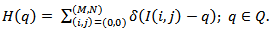(1)

Kronecker’s δ-function: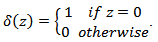(2)

Let’s build non-empty values of H(q)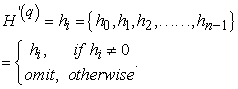(3)

Total number of each intensity’s frequency value will be less than L after removing zero frequency intensities,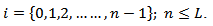(4)

In this stage, frequency values will be further reduced by combining neighboring frequency values and its corresponding intensity values if the difference between intensity values is equal to one.

For example, in Table 1, first two columns have intensity values and its frequencies. In level one operation, first two intensity values difference is one, so its corresponding frequency values of 125 and 35 are combined to 160. And it will be given single intensity value of 2 because it’s frequency values is higher than frequency of intensity 3. Similarly, frequency of intensity values of 11 and 12 are also combined to 729 and its intensity value is replaced with 12. Difference between next intensity values 14 and 22 are higher than one, so only it will remain the same values in its output. Next intensity values 22 and 23 are evaluated and so on until all remaining intensity values. In level 2 operation, difference between intensity values needs to be 2 or less and similar process is conducted to build level 2 columns. These levels of operations can be conducted until all intensity values will become single intensity value.

Let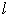be the level of operations, which is= [1,2,3…].

So the difference between intensity values will be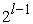= [1,2,4…]. Its new frequencies can be written as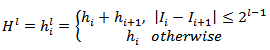(5)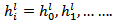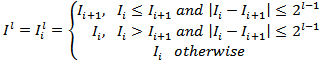(6)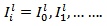High level of operation is chosen such that there is minimal changes on intensity values in next stage in order to increase PSNR values on its final preprocessed image.

In second stage, each pixel intensity from the original intensity values on first column sets is mapped to the closest intensity value on the level 1 columns sets (if level 1 operation is chosen to preprocess the image).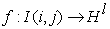for the image I maps to the closest value of intensity in Hl

If its value is one point away or closer to mapped intensity value, ±1 will be added to the pixel intensity value in Image I such that it will be equal or further close to the mapped intensity value. For example, if intensity value 122 and its closest map is equal to 118. Then the intensity value will be converted to 121. This is known as ±1 adjustment to the pixel intensity. This can be done for ±1, ±2, … called ±k adjustment. Image obtained from ±k adjustment is subjected to compression. For color images, this method is applied to each layer separately. In this paper, only results from gray scale image is presented.

3.1.1. Compression

The preprocessed files are compressed using Deflate, BZip2, LZMA 11 and 7zip software 13. Compression is implemented in Python 3.6 using zipfile module 11.

3.1.2. Performance of Preprocessing

We can estimate the performance of preprocessing by applying the quality measurement of the reconstructed image, and the compression ratio. Peak signal to noise ratio (PSNR) is used as a quality measurement metric and has a two-step calculation as shown below:

Mean Square Error (MSE) is a measure of the distortion rate in the reconstructed image.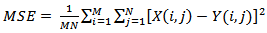(7)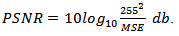(8)

The Compression ratio (CR) is the ratio between the original image size and the compressed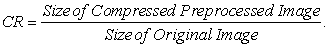(9)

### 4. Experimental Results

Source images were acquired from the SIPI image database maintained by the University of Southern California 12. Figure 7 shows the result of preprocessing grayscale image of a mandril, which is a large monkey. The first section Figure 2 is the histogram of the original image and it consists of 188 intensity values. These intensity values are reduced to 95, 48, 25, and 13 in Figure 2 -Figure 5. These are obtained by adding two frequencies of nearest intensity values. Nearest intensity value is 1 for first level (Figure 3), 4 for second level (Figure 4), 8 for third level (Figure 5), and 16 for fourth level (Figure 6). At higher level of operation, number of intensity values are less. Frequencies are used only for obtaining these intensity level. Level of operation is selected for higher PSNR values. If level of operation is 3, Figure 4 will be selected. Each pixel’s intensity from the Image is mapped to the closest intensity level in Figure 4. If it is 1 digit away or closer, then 1 digit is added/subtracted to the original image. So, at higher level, there is less chance for pixel to be closer to its value that leaves pixel unchanged. Instead of 1, it can be 2, 3 or more. But it will modify more pixels as more pixels will be closer intensities (Figure 5) losing PSNR. Table 1 shows the PSNR values at each level of operation by adjusting ±1, and its compressed size of preprocessed image is also presented.

Compressed size of unprocessed and preprocessed image of mandril is presented in Table 2 at six different level of operations. Compression ratio of unprocessed and preprocessed image is presented in Table 3. Table 4 and Table 5 presents the average of compressed size and compressed ratio from nineteen different images from SIPI Image Database 12 is presented.

• Figure 2. Histogram of Original Image
• Figure 3. Histogram of after Level 1 Operation
• Figure 4. Histogram of after Level 2 Operation
• Figure 5. Histogram of After Level 3 Operation
• Figure 6. Histogram of After Level 4 Operation
• Figure 7. Original Mandril Image
• Figure 8. Preprocessed Mandril Image at 3 level of operation
• Figure 9. Histogram of Preprocessed Mandril Image after level 1 operation
• Figure 10. Histogram of Preprocessed Mandril Image after level 6 operation

### 5. Conclusion

The results of this study proposes preprocessing techniques for high-efficiency image data compression. This is a two-stage preprocessing system. In the first stage, using histogram, intensity counts are reduced and mapping table identified by level of operation are created. In the second stage, each pixel’s intensity is mapped to the closest intensity of in the mapping table from first stage. Based on the distance to the closest pixel intensity value, pixel intensity values are adjusted by adding or subtracting one value (±1). Preprocessed images are compressed by using Deflate, Bzip2, LZMA, and 7zip methods. By performing this, it is possible to reduce the size of compressed file. From the results, it is possible to improve the compression ratio by 18% for Deflate algorithm at PSNR value of 54.85. For retaining more original information, higher level of operation can be selected at the cost of compression ratio. For more compression ratio, higher value in ±k adjustment can be selected at the cost of PSNR values. From the experimental results, it is shown that compression ratio can be improved by preprocessing image to the compressed images without preprocessing techniques.

### References

  P. Zahradnik, B. Simák, M. Vlcek, “Filter Design for Image Preprocessing in Image Communication,” in Eighth International Conference on Networks, 2009 (ICN09), pp. 40-45. 2009. In article View Article  F. Tushabe and M. H. F. Wilkinson, “Image preprocessing for compression: Attribute filtering,” in Proceedings of International Conference on Signal Processing and Imaging Engineering (ICSPIE’07), San Francisco, USA, October 2007, pp. 1411-1418. In article View Article  Milanova M., Kountchev R., Todorov V., Kountcheva R. “Pre- And Post-Processing for Enhancement Of Image Compression Based On Spectrum Pyramid,” in Sobh T., Elleithy K., Mahmood A., Karim M. (eds) Innovative Algorithms and Techniques in Automation, Industrial Electronics and Telecommunications. Springer, Dordrecht, pp. 269-274. 2007. In article View Article  Kwon, Y, Kim, KI & Kim, JH 2008. “Suppressing artifacts in block DCT coded images based on re-encoding, regression, and image prior,” in KAIST Department of Computer Science Technical Reports., CS-TR-2008-293. In article View Article  Ivan Kopilovic, Tamas Sziranyi, “Artifact reduction with diffusion preprocessing for image compression,” Optical Engineering, 44(2), 027003. 1.Feb. 2005. In article View Article  Krig S., Image Pre-Processing, Computer Vision Metrics, Apress, Berkeley, CA, 2014. In article PubMed  PubMed  J. Sauvola, H. Kauniskangas, K. Vainamo, “Automated document image preprocessing management utilizing grey-scale image analysis and neural network classification,” in Sixth International Conference on Image Processing and Its Applications, vol.2, pp. 502-506. Jul.1997. In article View Article  Sonka, M., Hlavac, V., Boyle, R., Image pre-processing: Image Processing Analysis and Machine Vision, Springer, New York, 1993. In article View Article  Balaji A., Sharma G., Shaw M.Q., Guay, R. “Preprocessing Methods for Improved Lossless Compression of Color Look-up Tables,” Journal of Imaging Science and Technology, SPIE – The International Society for Optical Engineering, vol. 52(4), 040901. 2008. In article View Article  J. Pinho, “An online preprocessing technique for improving the lossless compression of images with sparse histograms,” in IEEE Signal Processing Letters, vol. 9, no. 1, pp. 5-7, 2002. In article View Article  “13.5. zipfile - Work with ZIP archives – Python 3.6.5rc1 documentation,” [Online]. Available: https://docs.python.org/3.6/library/zipfile.html. [Accessed Sep.10, 2017]. In article View Article  “The USC-SIPI Image Database,” [Online]. Available: http://sipi.usc.edu/database. [Accessed Jun.12, 2017]. In article View Article  “7zip” [Online]. Available: https://7-zip.org. [Accessed Sep.10, 2017]. In article View ArticleThis work is licensed under a Creative Commons Attribution 4.0 International License. To view a copy of this license, visit http://creativecommons.org/licenses/by/4.0/